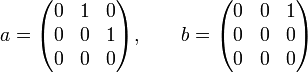# Algebra group structures for cyclic group:Z4

View algebra group structures for particular groups | View other specific information about cyclic group:Z4

There exists a unique way of making cyclic group:Z4 into an algebra group over field:F2. It is not an algebra group over any other field. This is unique in a strict sense, not merely up to isomorphisms.

## Summary

Algebra Smallest$n$ for which it can be embedded in$UT(n,2)$ Maximum of element nilpotencies Nilpotency index of whole algebra
the only possible one, where one generator squares to the other 3 3 3

## Algebra structure

### Multiplication table (structure constants)

The algebra is two-dimensional. It has the following multiplication table, with basis elements$a,b$. Note that Adjoint group of a radical ring is abelian iff the radical ring is commutative‎, so the algebra group is commutative, and we don't have to worry about the order of multiplication:$a$$b$$a$$b$ 0$b$ 0 0

### Verification of properties

•$N$ is associative: All products of length three or more involving basis elements are zero, hence, by linearity, all products of length three or more are zero.
•$N$ is nilpotent: All products of length three or more involving basis elements are zero, hence, by linearity, all products of length three or more are zero.
• The algebra group for$N$ is cyclic of order 4: Indeed,$(1 + a)^2 = 1 + b$ and$(1 + b)^2 = 1$, so$1 + a$ is an element of order four (see also powering map by field characteristic is same in algebra and algebra group).

### Description as subalgebra of niltriangular matrix Lie algebra

The algebra can be realized explicitly as a subalgebra of niltriangular matrix Lie algebra:NT(3,2) as follows:$a = \begin{pmatrix} 0 & 1 & 0 \\ 0 & 0 & 1 \\ 0 & 0 & 0 \\\end{pmatrix}, \qquad b = \begin{pmatrix} 0 & 0 & 1 \\ 0 & 0 & 0 \\ 0 & 0 & 0 \\\end{pmatrix}$